# Multiple Choice questions on heat and thermodynamics for JEE Main and Advanced

In this page we have Multiple Choice questions on heat and thermodynamics for JEE Main and Advanced . Hope you like them and do not forget to like , social shar and comment at the end of the page.

Question 1 The coefficient of linear expansion of an in homogenous rod changes linearly from α1to α2from one end to the other end of the rod. The effective coefficient of linear expansion of the rod is

a α12
b 1/2(α12)
c. √α1α2
d. (α12)

Question 2 An Aluminum Rod of length L0 rest on a smooth horizontal base if the temperature is increase by ΔT °C. What will be the longitudinal strain developed
a.     αΔT
b.     Zero
c.     -αΔT
d.     None of the above

Question 3 The ratio of adiabatic bulk modulus and isothermal bulk modulus of a gas (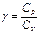)
a)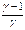b) 1
c)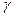d)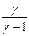Question 4  An animal of 70 kg is running with a speed of 6 m/s. If all the KE of animal can be used in increasing water from 18 °C to 32 °C, how much water can be heated with this energy

Question 5 A copper cube of mass 300g slides down on a rough inclined plane of inclination 40 ° at a constant speed. Assume that any loss in mechanical energy goes into copper block as thermal energy find the increase in thermal energy of the block as it slides down through 50 cm. specific heat capacity of copper = 420J/kg-k.
Question 6 It is known that curves A,B,C are Isobaric, Isothermal, Adiabatic process then when one is correct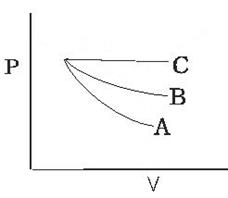a,     A - Adiabatic ,          B - Isothermal,,          C - Isobaric
b,     A- Isothermal,,          B - Adiabatic,          C - Isobaric
c,     A - Isobaric,          B - Isothermal          C - Adiabatic
d,     None of these

Question 7 A container has a mixture of 1 mole of oxygen and 2 moles of nitrogen at 330K.The ratio of average rotational kinetic energy per O2 molecule to that per H2 molecule is
a)  2:1
b) 1:2
c) 1:1
d) None of these

Question 8  The sprinkling of water reduces the temperature of the closed room
a) The water has large latent heat of vaporization
b) Water is bad conductor of heat
c) Specific heat of water is high
d) the temperature of water is less than that of room

Question 9 Two boxes A and B containing different ideal gases are placed on table
Box A contain one mole of gas m where (Cv=5R/2) at Temperature T0
Box B contains one mole of gas n where (Cv=3R/2) at temperature (7/3) T0
The boxes are then put into thermal contact with each other and heat flows between until the gases reach a common final temperature Tf
Which of the following relation is correct?
a) 2Tf-3T0=0
b) 2Tf-7T0=0
c) 2Tf-5T0=0
d) Tf-3T0=0

Question 10 During an adiabatic process the square of the pressure of a gas is proportional to the fifth power of its absolute temperature. The ratio of specific heat Cp / Cv for that gas is
a.      3/5
b.      4/3
c.      5/3
d.      3/2